# Modeling Probabilities of Default with Cox Proportional Hazards

This example shows how to work with consumer (retail) credit panel data to visualize observed probabilities of default (PDs) at different levels. It also shows how to fit a Cox proportional hazards (PH) model, also known as Cox regression, to predict PDs. In addition, it shows how to perform a stress-testing analysis, how to model lifetime PDs, and how to calculate the lifetime expected credit loss (ECL) value using `portfolioECL`.

This example uses `fitLifetimePDModel` from Risk Management Toolbox™ to fit the `Cox` PH model. Although the same model can be fitted using `fitcox`, the lifetime probability of default (PD) version of the `Cox` model is designed for credit applications, and supports conditional PD prediction, lifetime PD prediction, and model validation tools, including the discrimination and accuracy plots.

A similar example, Stress Testing of Consumer Credit Default Probabilities Using Panel Data, follows the same workflow, but it uses a `Logistic` regression model instead of a `Cox` model. The main differences in the two approaches are:

### Data Exploration with Survival Analysis Tools

Start with some data visualizations, mainly the visualization of PDs as a function of age, which in this data set is the same as years-on-books (YOB). Because `Cox` PH is a survival analysis model, this example discusses some survival analysis tools and concepts and uses the empirical cumulative distribution function (`ecdf`) functionality for some of these computations and visualizations.

The main data set (`data`) contains the following variables:

• `ID`: Loan identifier.

• `ScoreGroup`: Credit score at the beginning of the loan, discretized into three groups, `High Risk`, `Medium Risk`, and `Low Risk`.

• `YOB`: Years on books.

• `Default`: Default indicator. This is the response variable.

• `Year`: Calendar year.

There is also a small data set (`dataMacro`) with macroeconomic data for the corresponding calendar years that contains the following variables:

• `Year`: Calendar year.

• `GDP`: Gross domestic product growth (year over year).

• `Market`: Market return (year over year).

The variables `YOB`, `Year`, `GDP`, and `Market` are observed at the end of the corresponding calendar year. The `ScoreGroup` is a discretization of the original credit score when the loan started. A value of `1` for `Default` means that the loan defaulted in the corresponding calendar year.

A third data set (`dataMacroStress`) contains baseline, adverse, and severely adverse scenarios for the macroeconomic variables. The stress-testing analysis in this example uses this table.

```load RetailCreditPanelData.mat disp(head(data,10))```
``` ID ScoreGroup YOB Default Year __ ___________ ___ _______ ____ 1 Low Risk 1 0 1997 1 Low Risk 2 0 1998 1 Low Risk 3 0 1999 1 Low Risk 4 0 2000 1 Low Risk 5 0 2001 1 Low Risk 6 0 2002 1 Low Risk 7 0 2003 1 Low Risk 8 0 2004 2 Medium Risk 1 0 1997 2 Medium Risk 2 0 1998 ```

Preprocess the panel data to put it in the format expected by some of the survival analysis tools.

```% Use groupsummary to reduce data to one ID per row, and keep track of % whether the loan defaulted or not. dataSurvival = groupsummary(data,'ID','sum','Default'); disp(head(dataSurvival,10))```
``` ID GroupCount sum_Default __ __________ ___________ 1 8 0 2 8 0 3 8 0 4 6 0 5 7 0 6 7 0 7 8 0 8 6 0 9 7 0 10 8 0 ```
```% You can also get years observed from YOB, though in this example, the YOB always % starts from 1 in the data, so the GroupCount equals the final YOB. dataSurvival.Properties.VariableNames{2} = 'YearsObserved'; dataSurvival.Properties.VariableNames{3} = 'Default'; % If there is no default, it is a censored observation. dataSurvival.Censored = ~dataSurvival.Default; disp(head(dataSurvival,10))```
``` ID YearsObserved Default Censored __ _____________ _______ ________ 1 8 0 true 2 8 0 true 3 8 0 true 4 6 0 true 5 7 0 true 6 7 0 true 7 8 0 true 8 6 0 true 9 7 0 true 10 8 0 true ```

The main variable is the amount of time each loan was observed (`YearsObserved`), which is the final value of the years-on-books (`YOB`) variable. This years observed is the number of years until default, or until the end of the observation period (eight years), or until the loan is removed from the sample due to prepayment. In this data set, the `YOB` information is the same as the age of the loan because all loans start with a `YOB` of 1. For other data sets, this case might true. For example, in a trading portfolio, the `YOB` and age may be different because a loan purchased in the third year of its life would have an age of `3`, but a `YOB` value of 1.

The second required variable is the censoring variable (`Censored`). In this analysis, the event of interest is the loan default. If a loan is observed until default, you have all of the information about the time until default. Therefore, the lifetime information is uncensored or complete. Alternatively, the information is considered censored, or incomplete, if at the end of the observation period the loan has not defaulted. The loan could not default because it was prepaid or the loan had not defaulted by the end of the eight-year observation period in the sample.

Add the `ScoreGroup` and `Vintage` information to the data. The value of these variables remains constant throughout the life of the loan. The score given at origination determines the `ScoreGroup` and the origination year determines the `Vintage` or cohort.

```% You can get ScoreGroup from YOB==1 because, in this data set, % YOB always starts at 1 and the ID's order is the same in data and % dataSurvival. dataSurvival.ScoreGroup = data.ScoreGroup(data.YOB==1); % Define vintages based on the year the loan started. All loans % in this data set start in year 1 of their life. dataSurvival.Vintage = data.Year(data.YOB==1); disp(head(dataSurvival,10))```
``` ID YearsObserved Default Censored ScoreGroup Vintage __ _____________ _______ ________ ___________ _______ 1 8 0 true Low Risk 1997 2 8 0 true Medium Risk 1997 3 8 0 true Medium Risk 1997 4 6 0 true Medium Risk 1999 5 7 0 true Medium Risk 1998 6 7 0 true Medium Risk 1998 7 8 0 true Medium Risk 1997 8 6 0 true Medium Risk 1999 9 7 0 true Low Risk 1998 10 8 0 true Low Risk 1997 ```

Compare the number of rows in the original data set (in panel data format) and the aggregated data set (in the more traditional survival format).

`fprintf('Number of rows original data: %d\n',height(data));`
```Number of rows original data: 646724 ```
`fprintf('Number of rows survival data: %d\n',height(dataSurvival));`
```Number of rows survival data: 96820 ```

Plot the cumulative default probability against YOB for the entire portfolio (all score groups and vintages) using the empirical cumulative distribution function (`ecdf`).

```ecdf(dataSurvival.YearsObserved,'Censoring',dataSurvival.Censored,'Bounds','on') title('Cumulative Default Probability, All Score Groups') xlabel('Years on Books')```Plot conditional one-year PDs against YOB. For example, the conditional one-year PD for a `YOB` of `3` is the conditional one-year PD for loans that are in their third year of life. In survival analysis, this value coincides with the discrete hazard rate, denoted by h, since the number of defaults in a particular year is the number of "failures," and the number of loans still on books at the beginning of that same year is the same as the "number at risk." To compute h, get the cumulative hazard function output, denoted by H, and transform it to the hazard function h. For more information, see Kaplan-Meier Method.

```[H,x] = ecdf(dataSurvival.YearsObserved,'Censoring',dataSurvival.Censored, ... 'Function','cumulative hazard'); % Take the diff of H to get the hazard h. h = diff(H); x(1) = []; % In this example, the times observed (stored in variable x) do not change for % different score groups, or for training vs. test sets. For other data sets, % you may need to check the x and h variables after every call to the ecdf function before % plotting or concatenating results. (For example, if data set has no defaults in a % particular year for the test data.) plot(x,h,'*') grid on title('Conditional One-Year PDs') ylabel('PD') xlabel('Years on Books')```You can also compute these probabilities directly with `groupsummary` using the original panel data format. For more information, see the companion example, Stress Testing of Consumer Credit Default Probabilities Using Panel Data. Alternatively, you can compute these probabilities with `grpstats` using the original panel data format. Either of these approaches gives the same conditional one-year PDs.

```PDvsYOBByGroupsummary = groupsummary(data,'YOB','mean','Default'); PDvsYOBByGrpstats = grpstats(data.Default,data.YOB); PDvsYOB = table((1:8)',h,PDvsYOBByGroupsummary.mean_Default,PDvsYOBByGrpstats, ... 'VariableNames',{'YOB','ECDF','Groupsummary','Grpstats'}); disp(PDvsYOB)```
``` YOB ECDF Groupsummary Grpstats ___ _________ ____________ _________ 1 0.017507 0.017507 0.017507 2 0.012704 0.012704 0.012704 3 0.011168 0.011168 0.011168 4 0.010728 0.010728 0.010728 5 0.0085949 0.0085949 0.0085949 6 0.006413 0.006413 0.006413 7 0.0033231 0.0033231 0.0033231 8 0.0016272 0.0016272 0.0016272 ```

Segment the data by `ScoreGroup` to get the PDs disaggregated by `ScoreGroup`.

```ScoreGroupLabels = categories(dataSurvival.ScoreGroup); NumScoreGroups = length(ScoreGroupLabels); hSG = zeros(length(h),NumScoreGroups); for ii=1:NumScoreGroups Ind = dataSurvival.ScoreGroup==ScoreGroupLabels{ii}; H = ecdf(dataSurvival.YearsObserved(Ind),'Censoring',dataSurvival.Censored(Ind)); hSG(:,ii) = diff(H); end plot(x,hSG,'*') grid on title('Conditional One-Year PDs, By Score Group') xlabel('Years on Books') ylabel('PD') legend(ScoreGroupLabels)```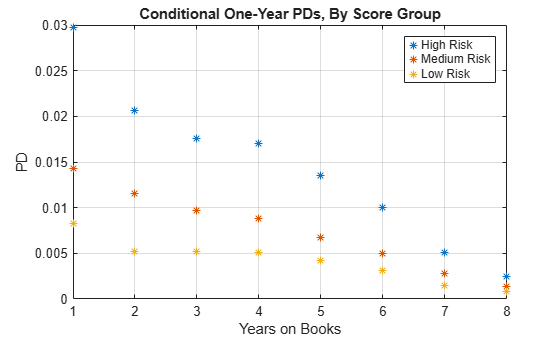You can also disaggregate PDs by `Vintage` information and segment the data in a similar way. You can plot these PDs against `YOB` or against calendar year. To see these visualizations, refer to Stress Testing of Consumer Credit Default Probabilities Using Panel Data.

### Cox PH Model Without Macro Effects

This section shows how to fit a `Cox` PH model without macro information. The model includes only the time-independent predictor `ScoreGroup` at the origination of the loans. Time-independent predictors contain information that remains constant throughout the life of the loan. This example uses only `ScoreGroup`, but other time-independent predictors could be added to the model (for example, `Vintage` information).

Cox proportional hazards regression is a semiparametric method for adjusting survival rate estimates to quantify the effect of predictor variables. The method represents the effects of explanatory variables as a multiplier of a common baseline hazard function, ${\mathit{h}}_{0}\left(\mathit{t}\right)$. The hazard function is the nonparametric part of the Cox proportional hazards regression function, whereas the impact of the predictor variables is a loglinear regression. The Cox PH model is:

`$\mathit{h}\left({\mathit{X}}_{\mathit{i}},\mathit{t}\right)={\mathit{h}}_{0}\left(\mathit{t}\right)\mathrm{exp}\left(\sum _{\mathit{j}=1}^{\mathit{p}}{\mathit{x}}_{\mathrm{ij}}{\mathit{b}}_{\mathit{j}}\right)$`

where:

• ${\mathit{X}}_{\mathit{i}}=\left({\mathit{x}}_{\mathrm{i1}},...,{\mathit{x}}_{\mathrm{ip}}\right)$ are the predictor variables for the ith subject.

• ${\mathit{b}}_{\mathit{j}}$ is the coefficient of the jth predictor variable.

• $\mathit{h}\left({\mathit{X}}_{\mathit{i}},\mathit{t}\right)$ is the hazard rate at time t for ${\mathit{X}}_{\mathit{i}}$.

• ${\mathit{h}}_{0}\left(\mathit{t}\right)$ is the baseline hazard rate function.

For more details, see the `Cox` and `fitcox` or Cox Proportional Hazards Model and the references therein.

The basic `Cox` PH model assumes that the predictor values do not change throughout the life of the loans. In this example, `ScoreGroup` does not change because it is the score given to borrowers at the beginning of the loan. `Vintage` is also constant throughout the life of the loan.

A `Cox` model could use time-dependent scores. For example, if the credit score information is updated every year, you model a time-dependent predictor in the `Cox` model similar to the way the macro variables are added to the model later in the Cox PH Model with Macro Effects section.

To fit a `Cox` lifetime PD model using `fitLifetimePDModel`, use the original `data` table in panel data format. Although the survival data format in the `dataSurvival` table can be used with other survival functions such as `ecdf` or `fitcox`, the `fitLifetimePDModel` function always works with the panel data format. This simplifies the switch between models with, or without time-dependent models, and the same panel data format is used for the validation functions such as `modelAccuracyPlot`. When fitting Cox models, the `fitLifetimePDModel` function treats the age variable (`'AgeVar'` argument) as the time to event and it uses the response variable (`'ResponseVar'` argument) binary values to identify the censored observations.

In the fitted model that follows, the only predictor is the `ScoreGroup` variable. The `fitLifetimePDModel` function checks the periodicity of the data (the most common age increments) and stores it in the `'TimeInterval'` property of the `Cox` lifetime PD model. The `'TimeInterval'` information is important for the prediction of conditional PD using `predict`.

Split the data into training and testing subsets and then fit the model using the training data.

```nIDs = max(data.ID); uniqueIDs = unique(data.ID); rng('default'); % For reproducibility c = cvpartition(nIDs,'HoldOut',0.4); TrainIDInd = training(c); TestIDInd = test(c); TrainDataInd = ismember(data.ID,uniqueIDs(TrainIDInd)); TestDataInd = ismember(data.ID,uniqueIDs(TestIDInd)); pdModel = fitLifetimePDModel(data(TrainDataInd,:),'cox', ... 'IDVar','ID','AgeVar','YOB','LoanVars','ScoreGroup','ResponseVar','Default'); disp(pdModel)```
``` Cox with properties: TimeInterval: 1 ExtrapolationFactor: 1 ModelID: "Cox" Description: "" Model: [1x1 CoxModel] IDVar: "ID" AgeVar: "YOB" LoanVars: "ScoreGroup" MacroVars: "" ResponseVar: "Default" ```
`disp(pdModel.Model)`
```Cox Proportional Hazards regression model: Beta SE zStat pValue ________ ________ _______ ___________ ScoreGroup_Medium Risk -0.67831 0.037029 -18.319 5.8806e-75 ScoreGroup_Low Risk -1.2453 0.045243 -27.525 8.8419e-167 ```

To predict the conditional PDs, use `predict`. For example, predict the PD for the first `ID` in the data.

`PD_ID1 = predict(pdModel,data(1:8,:))`
```PD_ID1 = 8×1 0.0083 0.0059 0.0055 0.0052 0.0039 0.0033 0.0016 0.0009 ```

To compare the predicted PDs against the observed default rates in the training or test data, use `modelAccuracyPlot`. This plot is a visualization of the accuracy of the predicted PD values (also known as model calibration, or predictive ability). A grouping variable is required for the PD model accuracy. By using `YOB` as the grouping variable, the observed default rates are the same as the default rates discussed in the Data Exploration with Survival Analysis Tools section.

```DataSetChoice ="Testing"; if DataSetChoice=="Training" Ind = TrainDataInd; else Ind = TestDataInd; end modelAccuracyPlot(pdModel,data(Ind,:),'YOB','DataID',DataSetChoice)```The accuracy plot accepts a second grouping variable. For example, use `ScoreGroup` as a second grouping variable to visualize the PD predictions per `ScoreGroup`, against the `YOB`.

`modelAccuracyPlot(pdModel,data(Ind,:),{'YOB','ScoreGroup'},'DataID',DataSetChoice)`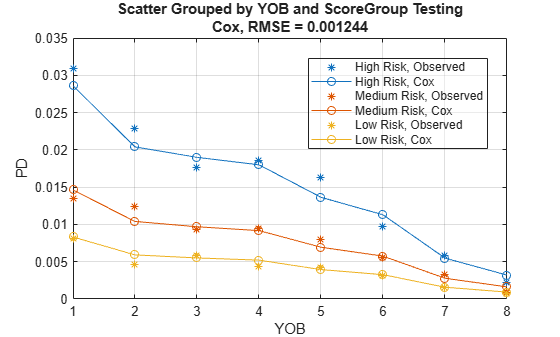The `modelDiscriminationPlot` returns the ROC curve. Use the optional `'SegmentBy'` argument to visualize the ROC for each `ScoreGroup`.

`modelDiscriminationPlot(pdModel,data(Ind,:),'DataID',DataSetChoice,'SegmentBy','ScoreGroup')`The nonparametric part of the Cox model allows it to closely match the training data pattern, even though only `ScoreGroup` is included as a predictor in this model. The results on test data show larger errors than on the training data, but this result is still a good fit.

The addition of macro information is important because both the stress testing and the lifetime PD projections require an explicit dependency on macro information.

### Cox PH Model with Macro Effects

This section shows how to fit a `Cox` PH model that includes macro information, specifically, gross domestic product (GDP) growth, and stock market growth. The value of the macro variables changes every year, so the predictors are time dependent.

The extension of the Cox proportional hazards model to account for time-dependent variables is:

`$\mathit{h}\left({\mathit{X}}_{\mathit{i}},\mathit{t}\right)={\mathit{h}}_{0}\left(\mathit{t}\right)\mathrm{exp}\left(\sum _{\mathit{j}=1}^{\mathrm{p1}}{\mathit{x}}_{\mathrm{ij}}{\mathit{b}}_{\mathit{j}}+\sum _{\mathit{k}=1}^{\mathrm{p2}}{\mathit{x}}_{\mathrm{i}\mathit{k}}\left(\mathit{t}\right){\mathit{c}}_{\mathit{k}}\right)$`

where:

• ${\mathit{x}}_{\mathrm{ij}}$ is the predictor variable value for the ith subject and the jth time-independent predictor.

• ${\mathit{x}}_{\mathrm{i}\mathit{k}}\left(\mathit{t}\right)$ is the predictor variable value for the ith subject and the kth time-dependent predictor at time t.

• ${\mathit{b}}_{\mathit{j}}$ is the coefficient of the jth time-independent predictor variable.

• ${\mathit{c}}_{\mathit{k}}$ is the coefficient of the kth time-dependent predictor variable.

• $\mathit{h}\left({\mathit{X}}_{\mathit{i}}\left(\mathit{t}\right),\mathit{t}\right)$ is the hazard rate at time t for ${\mathit{X}}_{\mathit{i}}\left(\mathit{t}\right).$

• ${\mathit{h}}_{0}\left(\mathit{t}\right)$ is the baseline hazard rate function.

For more details, see `Cox`, `fitcox`, or Cox Proportional Hazards Model and the references therein.

Macro variables are treated as time-dependent variables. If the time-independent information, such as the initial `ScoreGroup`, provides a baseline level of risk through the life of the loan, it is reasonable to expect that changing macro conditions may increase or decrease the risk around that baseline level. Also, if the macro conditions change, you can expect that these variations in risk will be different from one year to the next. For example, years with low economic growth should make all loans more risky, independently of their initial `ScoreGroup`.

The data input for the `Cox` lifetime PD model with time-dependent predictors uses the original panel data with the addition of the macro information.

As mentioned earlier, when fitting Cox models, the `fitLifetimePDModel` function treats the age variable (`'AgeVar'` argument) as the time to event and it uses the response variable (`'ResponseVar'` argument) binary values to identify the censored observations. In the fitted model that follows, the predictors are `ScoreGroup`, `GDP`, and `Market`. The `fitLifetimePDModel` checks the periodicity of the data (the most common age increments) and stores it in the `'TimeInterval'` property of the `Cox` lifetime PD model. For time-dependent models, the `'TimeInterval'` value is used to define age intervals for each row where the predictor values are constant. For more information, see Time Interval for Cox Models. The `'TimeInterval'` information is also important for the prediction of conditional PD when using `predict`.

Internally, the `fitLifetimePDModel` function uses `fitcox`. Using `fitLifetimePDModel` for credit models offers some advantages over `fitcox`. For example, when you work directly with `fitcox`, you need a survival version of the data for time-independent models and a "counting process" version of the data (similar to the panel data form, but with additional information) is needed for time-dependent models. The `fitLifetimePDModel` function always takes the panel data form as input and peforms the data preprocessing before calling `fitcox`. Also, with the lifetime PD version of the `Cox` model, you have access to credit-specific prediction and validation functionality not directly supported in the underlying Cox model.

```data = join(data,dataMacro); head(data)```
```ans=8×7 table ID ScoreGroup YOB Default Year GDP Market __ __________ ___ _______ ____ _____ ______ 1 Low Risk 1 0 1997 2.72 7.61 1 Low Risk 2 0 1998 3.57 26.24 1 Low Risk 3 0 1999 2.86 18.1 1 Low Risk 4 0 2000 2.43 3.19 1 Low Risk 5 0 2001 1.26 -10.51 1 Low Risk 6 0 2002 -0.59 -22.95 1 Low Risk 7 0 2003 0.63 2.78 1 Low Risk 8 0 2004 1.85 9.48 ```
```pdModelMacro = fitLifetimePDModel(data(TrainDataInd,:),'cox', ... 'IDVar','ID','AgeVar','YOB','LoanVars','ScoreGroup', ... 'MacroVars',{'GDP','Market'},'ResponseVar','Default'); disp(pdModelMacro)```
``` Cox with properties: TimeInterval: 1 ExtrapolationFactor: 1 ModelID: "Cox" Description: "" Model: [1x1 CoxModel] IDVar: "ID" AgeVar: "YOB" LoanVars: "ScoreGroup" MacroVars: ["GDP" "Market"] ResponseVar: "Default" ```
`disp(pdModelMacro.Model)`
```Cox Proportional Hazards regression model: Beta SE zStat pValue __________ _________ _______ ___________ ScoreGroup_Medium Risk -0.6794 0.037029 -18.348 3.4442e-75 ScoreGroup_Low Risk -1.2442 0.045244 -27.501 1.7116e-166 GDP -0.084533 0.043687 -1.935 0.052995 Market -0.0084411 0.0032221 -2.6198 0.0087991 ```

Visualize the accuracy (also known as model calibration, or predictive ability) of the predicted PD values using `modelAccuracyPlot`.

```DataSetChoice ="Testing"; if DataSetChoice=="Training" Ind = TrainDataInd; else Ind = TestDataInd; end modelAccuracyPlot(pdModelMacro,data(Ind,:),'YOB','DataID',DataSetChoice)```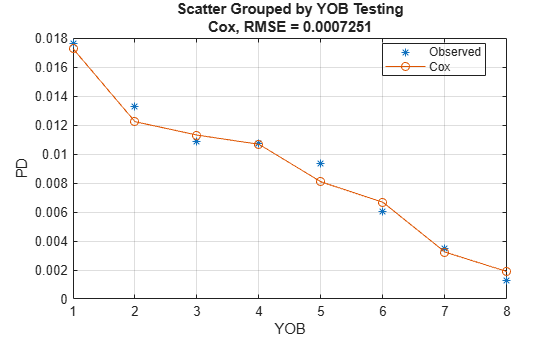The macro effects help the model match the observed default rates even closer and the match to the training data looks like an interpolation for the macro model.

The accuracy plot by `ScoreGroup` and the ROC curve is created the same way as for the Cox model without macro variables.

### Stress Testing

This section shows how to perform a stress-testing analysis of PDs using the `Cox` macro model.

Assume that a regulator has provided the following stress scenarios for the macroeconomic variables `GDP` and `Market`.

`disp(dataMacroStress)`
``` GDP Market _____ ______ Baseline 2.27 15.02 Adverse 1.31 4.56 Severe -0.22 -5.64 ```

The following code predicts PDs for each `ScoreGroup` and each macro scenario. For the visualization of each macro scenario, take the average over the `ScoreGroups` to aggregate the data into a single PD by `YOB`.

```dataStress = table; dataStress.YOB = repmat((1:8)',3,1); dataStress.ScoreGroup = repmat("",size(dataStress.YOB)); dataStress.ScoreGroup(1:8) = ScoreGroupLabels{1}; dataStress.ScoreGroup(9:16) = ScoreGroupLabels{2}; dataStress.ScoreGroup(17:24) = ScoreGroupLabels{3}; dataStress.GDP = zeros(size(dataStress.YOB)); dataStress.Market = zeros(size(dataStress.YOB)); ScenarioLabels = dataMacroStress.Properties.RowNames; NumScenarios = length(ScenarioLabels); PDScenarios = zeros(length(x),NumScenarios); for jj=1:NumScenarios Scenario = ScenarioLabels{jj}; dataStress.GDP(:) = dataMacroStress.GDP(Scenario); dataStress.Market(:) = dataMacroStress.Market(Scenario); % Predict PD for each ScoreGroup for the current scenario. dataStress.PD = predict(pdModelMacro,dataStress); % Average PD over ScoreGroups, by age, to visualize in a single plot. PDAvgTable = groupsummary(dataStress,"YOB","mean","PD"); PDScenarios(:,jj) = PDAvgTable.mean_PD; end figure; bar(x,PDScenarios) title('Stress Test, Probability of Default') xlabel('Years on Books') ylabel('PD') legend('Baseline','Adverse','Severe') grid on```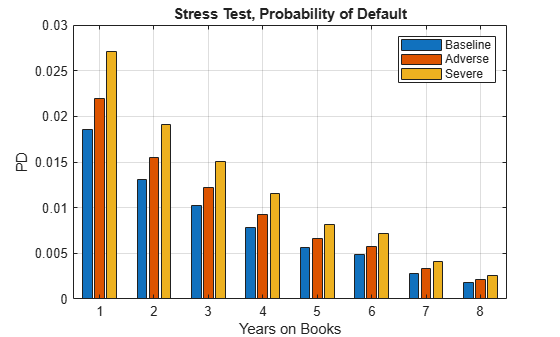This section shows how to compute lifetime PDs using the `Cox` macro model and how to compute lifetime expected credit losses (ECL).

For lifetime modeling, the PD model is the same, but it is used differently. You need the predicted PDs not just one period ahead, but for each year throughout the life of each particular loan. You also need macro scenarios throughout the life of the loans. This example sets up alternative long-term macro scenarios, computes lifetime PDs under each scenario, and computes the corresponding one-year PDs, marginal PDs, and survival probabilities. The lifetime and marginal PDs are visualized for each year, under each macro scenario. The ECL is then computed for each scenario and the weighted average lifetime ECL.

For concreteness, this example looks into an eight-year loan at the beginning of its third year and predicts the one-year PD from years `3` through `8` of the life of this loan. This example also computes the survival probability over the remaining life of the loan. The relationship between the survival probability $\mathit{S}\left(\mathit{t}\right)$ and the one-year conditional PDs or hazard rates $\mathit{h}\left(\mathit{t}\right)$, sometimes also called the forward PDs, is:

`$\begin{array}{l}\mathit{S}\left(0\right)=1,\\ \mathit{S}\left(1\right)=\left(1-\mathrm{PD}\left(1\right)\right),\\ ...\\ \mathit{S}\left(\mathit{t}\right)=\mathit{S}\left(\mathit{t}-1\right)\left(1-\mathrm{PD}\left(\mathit{t}\right)\right)=\left(1-\mathrm{PD}\left(1\right)\right)\cdots \left(1-\mathrm{PD}\left(\mathit{t}\right)\right)\end{array}$`

The lifetime PD (LPD) is the cumulative PD over the life of the loan, given by the complement of the survival probability:

`$\mathrm{LPD}\left(\mathit{t}\right)=1-\mathit{S}\left(\mathit{t}\right)$`

Another quantity of interest is the marginal PD (MPD), which is the increase in the lifetime PD between two consecutive periods:

`$\mathrm{MPD}\left(\mathit{t}+1\right)=\mathrm{LPD}\left(\mathit{t}+1\right)-\mathrm{LPD}\left(\mathit{t}\right)$`

It follows that the marginal PD is also the decrease in survival probability between consecutive periods, and also the hazard rate multiplied by the survival probability:

`$\mathrm{MPD}\left(\mathit{t}+1\right)=\mathit{S}\left(\mathit{t}\right)-\mathit{S}\left(\mathit{t}+1\right)=\mathrm{PD}\left(\mathit{t}+1\right)\mathit{S}\left(\mathit{t}\right)$`

For more information, see `predictLifetime` and Kaplan-Meier Method. The `predictLifetime` function supports lifetime PD, marginal PD, and survival probability formats.

Specify three macroeconomic scenarios, one baseline projection, and two simple shifts of 20% higher or 20% lower values for the baseline growth, which are called faster growth and slower growth, respectively. The scenarios in this example, and the corresponding probabilities, are simple scenarios for illustration purposes only. A more thorough set of scenarios can be constructed with more powerful models using Econometrics Toolbox™ or Statistics and Machine Learning Toolbox™; see, for example, Modeling the United States Economy (Econometrics Toolbox). Automated methods can usually simulate large numbers of scenarios. In practice, only a small number of scenarios are required and these scenarios, and their corresponding probabilities, are selected combining quantitative tools and expert judgment. Also, see the Economic Scenarios and Expected Credit Loss Calculations example that shows a detailed workflow for ECL calculations, including the determination of macro scenarios.

```CurrentAge = 3; % Currently starting third year of loan Maturity = 8; % Loan ends at end of year 8 YOBLifetime = (CurrentAge:Maturity)'; NumYearsRemaining = length(YOBLifetime); dataLifetime = table; dataLifetime.ID = ones(NumYearsRemaining,1); dataLifetime.YOB = YOBLifetime; dataLifetime.ScoreGroup = repmat("High Risk",size(dataLifetime.YOB)); % High risk dataLifetime.GDP = zeros(size(dataLifetime.YOB)); dataLifetime.Market = zeros(size(dataLifetime.YOB)); % Macro scenarios for lifetime analysis GDPPredict = [2.3; 2.2; 2.1; 2.0; 1.9; 1.8]; GDPPredict = [0.8*GDPPredict GDPPredict 1.2*GDPPredict]; MarketPredict = [15; 13; 11; 9; 7; 5]; MarketPredict = [0.8*MarketPredict MarketPredict 1.2*MarketPredict]; ScenLabels = ["Slower growth" "Baseline" "Faster growth"]; NumMacroScen = size(GDPPredict,2); % Scenario probabilities for the computation of lifetime ECL PScenario = [0.2; 0.5; 0.3]; PDLifetime = zeros(size(GDPPredict)); PDMarginal = zeros(size(GDPPredict)); for ii = 1:NumMacroScen dataLifetime.GDP = GDPPredict(:,ii); dataLifetime.Market = MarketPredict(:,ii); PDLifetime(:,ii) = predictLifetime(pdModelMacro,dataLifetime); % Returns lifetime PD by default PDMarginal(:,ii) = predictLifetime(pdModelMacro,dataLifetime,'ProbabilityType','marginal'); end % Start lifetime PD at last year with value of 0 for visualization % purposes. tLifetime0 = (dataMacro.Year(end):dataMacro.Year(end)+NumYearsRemaining)'; PDLifetime = [zeros(1,NumMacroScen);PDLifetime]; tLifetime = tLifetime0(2:end); figure; subplot(2,1,1) plot(tLifetime0,PDLifetime) xticks(tLifetime0) grid on xlabel('Year') ylabel('Lifetime PD') title('Lifetime PD by Scenario') legend(ScenLabels,'Location','best') subplot(2,1,2) bar(tLifetime,PDMarginal) grid on xlabel('Year') ylabel('Marginal PD') title('Marginal PD by Scenario') legend(ScenLabels)```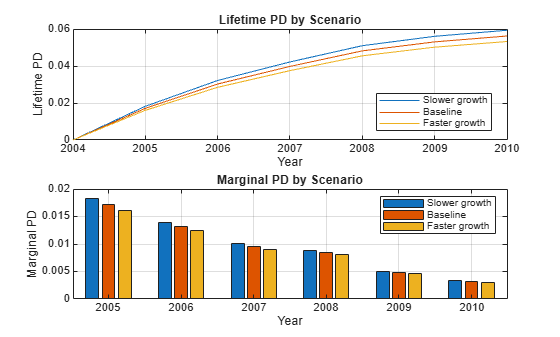These lifetime PDs, by scenario, are one of the inputs for the computation of lifetime expected credit losses (ECL). ECL also requires lifetime values for loss given default (LGD) and exposure at default (EAD), for each scenario, and the scenario probabilities. For simplicity, this example assumes a constant LGD and EAD value, but these parameters for LGD and EAD models could vary by scenario and by time period. For more information, see `fitLGDModel` and `fitEADModel`. Use `portfolioECL` to compute the lifetime ECL.

The computation of lifetime ECL also requires the effective interest rate (EIR) for discounting purposes. In this example, the discount factors are computed at the end of the time periods, but other discount times may be used. For example, you might use the midpoint in between the time periods; that is, discount first-year amounts with a 6-month discount factor, discount second-year amounts with a 1.5-year discount factor, and so on).

With these inputs, the expected credit loss at time t for scenario s is defined as:

`$\mathrm{ECL}\left(\mathit{t};\mathit{s}\right)=\mathrm{MPD}\left(\mathit{t};\mathit{s}\right)\text{\hspace{0.17em}}\mathrm{LGD}\left(\mathit{t};\mathit{s}\right)\text{\hspace{0.17em}}\mathrm{EAD}\left(\mathit{t};\mathit{s}\right)\text{\hspace{0.17em}}\mathrm{Disc}\left(\mathit{t}\right)$`

where t denotes a time period, s denotes a scenario, and $\mathrm{Disc}\left(\mathit{t}\right)=\frac{1}{{\left(1+\mathrm{EIR}\right)}^{\mathit{t}}}$.

For each scenario, a lifetime ECL is computed by adding ECLs across time, from the fist time period in the analysis, to the expected life of the product denoted by T. In this example, it is five years (this loan is a simple loan with five years remaining to maturity):

`$\mathrm{ECL}\left(\mathit{s}\right)=\sum _{\mathit{t}=1}^{\mathit{T}}\mathrm{ECL}\left(\mathit{t};\mathit{s}\right)$`

Finally, compute the weighed average of these expected credit losses, across all scenarios, to get a single lifetime ECL value, where $\mathit{P}\left(\mathit{s}\right)$ denotes the scenario probabilities:

`$\mathrm{ECL}=\sum _{\mathit{s}=1}^{\mathrm{NumScenarios}}\mathrm{ECL}\left(\mathit{s}\right)\mathit{P}\left(\mathit{s}\right)$`

These computations are supported with the `portfolioECL` function.

```LGD = 0.55; % Loss given default EAD = 100; % Exposure at default EIR = 0.045; % Effective interest rate PDMarginalTable = table(dataLifetime.ID, PDMarginal(:,1), PDMarginal(:,2), PDMarginal(:,3),'VariableNames',["ID",ScenLabels]); LGDTable = table(dataLifetime.ID(1), LGD,'VariableNames',["ID","LGD"]); EADTable = table(dataLifetime.ID(1), EAD,'VariableNames',["ID","EAD"]); [totalECL, ECLByID, ECLByPeriod] = portfolioECL(PDMarginalTable, LGDTable, EADTable, 'InterestRate', EIR,... 'ScenarioNames',ScenLabels, 'ScenarioProbabilities',PScenario, 'IDVar','ID','Periodicity','annual'); disp(ECLByID);```
``` ID ECL __ ______ 1 2.7441 ```
`disp(ECLByPeriod);`
``` ID TimePeriod Slower growth Baseline Faster growth __ __________ _____________ ________ _____________ 1 1 0.95927 0.90012 0.8446 1 2 0.703 0.66366 0.62646 1 3 0.48217 0.45781 0.43463 1 4 0.40518 0.38686 0.36931 1 5 0.22384 0.21488 0.20624 1 6 0.13866 0.13381 0.1291 ```
`fprintf('Lifetime ECL: %g\n',totalECL)`
```Lifetime ECL: 2.7441 ```

When the LGD and EAD do not depend on the scenarios (even if they change with time), the weighted average of the lifetime PD curves is taken to get a single, average lifetime PD curve.

```MarginalPDLifetimeWeightedAvg = PDMarginal*PScenario; MarginalPDLifetimeWeightedAvgTable = table(dataLifetime.ID, MarginalPDLifetimeWeightedAvg,'VariableNames',["ID","PD"]); totalECLByWeightedPD = portfolioECL(MarginalPDLifetimeWeightedAvgTable, LGDTable, EADTable, 'InterestRate', EIR,... 'IDVar','ID','Periodicity','annual'); fprintf('Lifetime ECL, using weighted lifetime PD: %g, same result because of constant LGD and EAD.\n', ... totalECLByWeightedPD)```
```Lifetime ECL, using weighted lifetime PD: 2.7441, same result because of constant LGD and EAD. ```

However, when the LGD and EAD values change with the scenarios, pass scenario-dependent inputs (the `PDMarginalTable` input) to the `portfolioECL` function to first compute the ECL values at scenario level. Then you can find the weighted average of the ECL values. For example, see Economic Scenarios and Expected Credit Loss Calculations where all inputs (marginal PD, LGD and EAD) change period-by-period and are sensitive to the macroeconomic scenarios.

### Conclusion

This example showed how to fit a Cox model for PDs, how to perform stress testing of the PDs, and how to compute lifetime PDs and ECL. A similar example, Stress Testing of Consumer Credit Default Probabilities Using Panel Data, follows the same workflow but uses logistic regression, instead of Cox regression. The `fitLifetimePDModel` function supports `Cox`, `Logistic`, and `Probit` models. The computation of lifetime PDs and ECL at the end of this example can also be performed with logistic or probit models. For an example, see Expected Credit Loss Computation.

### References

 Baesens, Bart, Daniel Roesch, and Harald Scheule. Credit Risk Analytics: Measurement Techniques, Applications, and Examples in SAS. Wiley, 2016.

 Bellini, Tiziano. IFRS 9 and CECL Credit Risk Modelling and Validation: A Practical Guide with Examples Worked in R and SAS. San Diego, CA: Elsevier, 2019.

 Federal Reserve, Comprehensive Capital Analysis and Review (CCAR): https://www.federalreserve.gov/bankinforeg/ccar.htm

 Bank of England, Stress Testing: https://www.bankofengland.co.uk/financial-stability

 European Banking Authority, EU-Wide Stress Testing: https://www.eba.europa.eu/risk-analysis-and-data/eu-wide-stress-testing